August 2022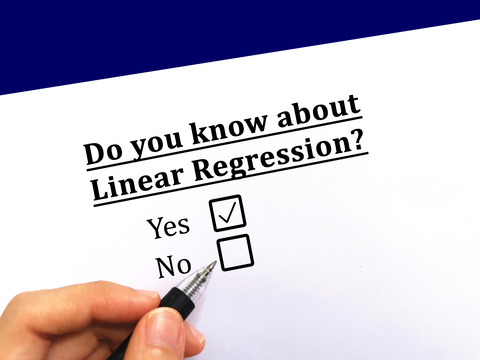Linear regression is often used to build a model where one or more predictor variables (the Xs) can be used to predict the response variable (Y). You end up with a model, which is an equation that describes the response variable in terms of the predictor variables. The predictor variables are the independent variables in statistical terms, while the response variable is the dependent variable.

Software makes it easy to run regression analysis. But software also has the capability to generate a lot of other regression statistics beyond the model – all designed to help decide how "good" the model is. This publication examines these regression statistics – what they mean and how do they help you understand how useful the model is. These terms include R2, PRESS, adjusted R2, VIF, standardized coefficients and much more.

The second part of this publication will focus on the different types of residuals and what they mean in regression analysis.

In this issue:

You may download a workbook containing the example data and regression results used below at this link. This workbook shows how all the regression statistics used in this publication are calculated. It also shows the regression output from the SPC for Excel software.

### Example Data

The data and much of the information contained in this publication comes from the book "Introduction to Linear Regression Analysis" by Douglas Montgomery, Elizabeth Peck, and Geoffrey Vining. This is an excellent reference on linear regression analysis. The example used below is the delivery time data in the book. A soft drink distributor wants to predict the amount of time (y) a delivery driver will take to service vending machines. There are two predictor variables that he is interested in exploring: the number of cases of product stocked (x1) and the distance walked in feet by the delivery driver (x2).

The data are given in Table 1. There are a total of 25 observations (delivery trips). For each trip, the number of cases stocked, the distance walked in feet, and the time taken in minutes were recorded.

Table 1: Delivery Time Data

Observation Number Number of Cases Distance Delivery Time   Observation Number Number of Cases Distance Delivery Time
1 7 560 16.68   14 6 462 19.75
2 3 220 11.5   15 9 448 24
3 3 340 12.03   16 10 776 29
4 4 80 14.88   17 6 200 15.35
5 6 150 13.75   18 7 132 19
6 7 330 18.11   19 3 36 9.5
7 2 110 8   20 17 770 35.1
8 7 210 17.83   21 10 140 17.9
9 30 1460 79.24   22 26 810 52.32
10 5 605 21.5   23 9 450 18.75
11 16 688 40.33   24 8 635 19.83
12 10 215 21   25 4 150 10.75
13 4 255 13.5

A multiple linear regression was performed using these data and the SPC for Excel software. The regression output generated are explained below.

### Regression Model

The purpose of regression analysis, of course, is to generate a model that predicts the response variable (delivery time) from the values of the predictor variables (number of cases and the distance walked). The form of the model is:

y = b0 + b1x1 + b2x2

where y is the response variable (delivery time), b0 is the intercept, b1 is the coefficient for x1 (number of cases) and b2 is the coefficient for x2 (distance). The model from the SPC for Excel analysis is given below.

Delivery Time = 2.341 + 1.616(Number of Cases) + 0.0144(Distance)

The model allows the delivery time to be predicted by inserting the number of cases and the distance into the equation. For example, if the number of cases is 25 and the distance walked is 100 feet, the delivery time is predicted to be:

Delivery Time = 2.341 + 1.616(25) + 0.0144(100) = 44.18

The coefficients represent how much time is added per unit change in the coefficient. For example, b1, = 1.616. This means that each additional case added increases the delivery time by 1.616 minutes on average.

You can use this model to predict the delivery time based on the number of cases and the distance. However, there is much we don't know just by looking at this model. For example, are the predictor variables statistically significant, i.e., do they really add something to the model. How much of the variation in delivery time is explained by the predictor variables in the model? Which predictor variable has the most impact? This is where the rest of the regression output comes in. These statistics help you answer these types of questions.

### Regression ANOVA Table

Analysis of Variance (ANOVA) is used to determine if the linear regression is significant. This ANOVA is testing the following hypothesis (using just two factors), where H0 is the null hypothesis and H1 is the alternate hypothesis:

H0: β1 = β2 = 0

H1: βi ≠ 0 for at least one i

where βi is the coefficient of predictor variable i. If you reject the null hypothesis, then at least one of the predictor variables contributes significantly to the model, meaning it has a significant effect on the delivery time.

The ANOVA table for the delivery example is shown below.

Table 2: ANOVA for Delivery Time

Source df SS MS F p value
Model 2 5550.8 2775.4 261.24 0.0000
Residual 22 233.7 10.62
Total 24 5784.5

The first column lists the three sources of variation: model, residual and total. This is how the variation is partitioned in the linear regression. The remaining columns are explained below.

SS: This is the sum of squares columns. The sum of squares is a measure of variation, measuring the sum of square differences as shown below. It is also used to determine how well the data fits the model generated by linear regression. The ANOVA table divides the total sum of squares from the regression analysis into two parts: the model (or regression) sum of squares and the residual sum of squares.

The total sum of squares is given by: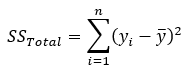where yi is the ith observation and y is the average of all the observations. From the equation, you can see that the total sum of squares is the sum of the squared deviations of each observation from the average. It represents the total variation in the observations. The total sum of squares for the delivery example is 5784.5.

The model or regression sum of squares describes how well the model fits the data. The model sum of squares is given by: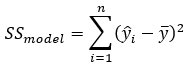where y ̂i is the predicted value of the ith observation. From the equation, you can see it is the sum of the square deviations between the predicted value based on the model and the average of the observations. The model sum of squares for the delivery example is 5550.8. Note that this is close to the total sum of squares. This implies that the model explains much of the total variation.

The residual sum of squares compares the predicted value for each observation to the observed value – again using the squared deviations. The residual sum of squares measures how much variability is unaccounted for by the model. The residuals sum of square is often referred to as the sum of squares for the error. The residual sum of squares is given by: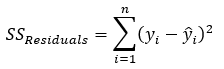The value for the residual sum of squares for the delivery example is 233.7. Note that this is small compared to the total sum of squares – another indication that the model explains much of the total variation.

Note: if you manually do the calculations using the model above, you will get slightly different values due to rounding of the coefficients.

df: This is the degrees of freedom for each source of variation. It defines the number of values in a dataset having the freedom to vary. If p = the number of parameters in the model and n = the number of observations, then the degrees of freedom for each source is given by:

• Model df = p
• Residual df = n - p - 1
• Total df = n - 1

In the delivery example, n = 25 and p = 2, so the degrees of freedom are 2, 22, and 24 for the model, the residuals, and the total, respectively.

MS: This is the mean square column. The mean square is an estimate of the variance for each source. It is calculated by dividing the sum of squares by the degrees of freedom for each source:

MS = SS/df

The mean squares are used in regression to determine which predictor variables are significant. The mean square for the residuals is called the mean square error (MSE). MSE is given by:

MSE = MSResiduals = SSResiduals/dfResiduals

The values for the mean squares in the delivery example are 2775.4 and 10.62 for the model and error respectively.

F: This is the F-value which is given by:

F = MSModel/MSE

This F value is used to assign a probability that the model is significant. The F value for the delivery example is 261.24.

p Value: This is the probability that you will get the F value above if the null hypothesis is true. If the p value is small, then you reject the null hypothesis and say that the model is statistically significant. In general, you can interpret the p value as:

• If the p-value is <=0.05, it is assumed that the model is statistically significant.
• If the p-value is above 0.20, it is assumed that the model is not statistically significant.
• If the p-value is between 0.05 and 0.20, the model may or may not be statistically significant.

In Excel, you can calculate the p value using the FDIST as shown below:

p Value = FDIST(F, dfModel, dfError)

which returns the right-tail F probability distribution. The p value for the delivery example is essentially 0 in Table 2. Since this value is less than 0.05, you conclude that the model is statistically significant. One or both predictor variables significantly impact the delivery time.

### Predictors Table

The predictors table contains the results for the coefficients and the intercept. The Predictors Table is shown in Table 3.

Table 3: Predictors Table

Coeff. Standard Error t Stat p Value 95% Lower 95% Upper VIF Stand. Coeff
Intercept 2.341 1.097 2.135 0.0442 0.0668 4.616
Number of Cases 1.616 0.171 9.464 0.0000 1.262 1.970 3.118 0.716
Distance 0.0144 0.00361 3.981 0.0006 0.00689 0.0219 3.118 0.301

The table contains the following:

Coefficient: These are the intercept and the coefficients for the predictor factors; the coefficients can be found using matrix algebra:

β = (X'X)-1X'Y

The β vector contains the coefficients.  The "prime" is the transposed matrix and the "-1" is the inverse matrix. The workbook mentioned above shows how these matrix calculations are done in Excel.

Standard Error: This is a measure of the precision of the coefficient estimates given above. The smaller the standard error, the more precise the coefficient estimate is. This standard error will help determine if the coefficient is statistically significant, i.e., not equal to 0. The standard error for coefficient i, se(Bi), is given by: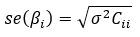where σ2 is the mean square residual or error (as shown in Table 2) and Cii is the diagonal elements of the (X'X)-1 matrix.

t Stat: This is the t statistic (from the t distribution) that is used to build a confidence interval around each coefficient estimate. If the confidence interval does not contain 0, then the coefficient is statistically significant. If the confidence interval does contain 0, then the coefficient is not statistically significant. The t statistic, t0, is the coefficient estimate divided by the standard error for the coefficient: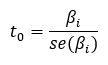p Value: The p value is the probability of getting the value of the t statistic if the null hypothesis is true. You can use the interpretation of the p value given above. Here, you are applying the p value to each coefficient. To determine the p value, you can use the T.Dist.2T function in Excel. It has the following form:

p value = T.Dist.2T(|t0|, dfResiduals)

Table 3 shows that each p value is less than 0.05. This means that each coefficient statistically impacts the delivery time.

95% Lower and Upper Confidence Limits: This is the 95% confidence interval for the coefficient estimate. If the interval contains 0, the null hypothesis is accepted. If it does not contain 0, the null hypothesis is rejected. The value of alpha is 0.05, which gives 95% confidence limits. The confidence interval is given by: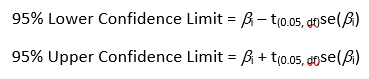where t is the value of the t distribution for alpha = 0.05 and the residual degrees of freedom. You can use T.INV.2T in Excel to determine the value of t. You can see from Table 3, that none of the intervals for the intercept, the number of cases, or the distance contain 0. This means that each are statistically significant.

VIF: This is the variance inflation factor which measures the multi-collinearity (the correlation between predictor variables). If there is a large correlation between the predictor variables, the estimate of regression coefficients will be dramatically impacted. The values of VIF are determined as follows: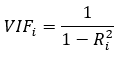where Ri2 is the R squared value when regressing xi on the other predictor variables. R squared is described in more detail below. If the other predictor variables predict xi well, the value of R squared will be close to 1, leading to a large VIF value. The interpretation of VIF is given below.

• VIF = 1, no correlation
• 1 < VIF < 5, moderate correlation
• 5 < VIF < 10, high correlation
• VIF > 10, may be impacting the regression analysis

In the delivery example, VIF is 3.118 for both predictor variables. This means that there is no significant correlation between the two predictor variables to impact the regression analysis.

Stand. Coeff: This gives the standardized regression coefficients. These are dimensionless coefficients that give you an estimate of the relative impact of each coefficient on the response variable. The coefficient estimates, given in the predictors table, reflect the unit of measure for each predictor variable. Because of this, you can't simply compare the coefficients for the predictor variables to determine which has the greater impact on y. But you can directly compare the standardized coefficients to determine which have the greatest impact. The standardized coefficients are given by the following: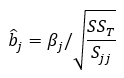where b ̂j is the standardized coefficient for predictor variable j, βj is the original coefficient, SST is the total sum of squares, and Sjj is given by: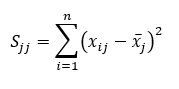n is the number of points and x represents a predictor variable. From Table 3, the standardized coefficients for the number of cases and distance are 0.716 and 0.301 respectively. This means that the number of cases has a larger impact on delivery time than distance.

### Regression Statistics Table

The regression statistics table is shown in Table 4.

Table 4: Regression Statistics

Statistic
R-sq 95.96%
Mean 22.38
Standard Error 3.259
Coefficient of Variation 14.56
Observations 25
Durbin-Watson Statistic 1.170
PRESS 459.0
R-sq Prediction 92.06%

The table contains the following:

R-sq: This is the R squared (or R2) value. It is called the coefficient of determination. It gives the amount of variability in the y values that is accounted for by the predictor variables. R2 is given by: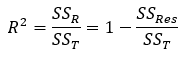where SSR is the model sum of squares, SSRes is the residual sum of squares, and SST is the total sum of squares. The following is true for R2:

0 ≤ R2 ≤ 1

In the delivery example, R2 = 95.96%. This means that 95.96% of the variation in delivery time is explained by the model.

Adjusted R-sq: This is the adjusted R2 value, which is given by: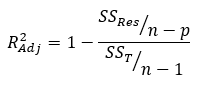where p = the number of coefficients. You use the adjusted R2 value to compare models that have different number of predictor variables. As you add more predictor variables to the model, the R2 value will always increase.

This is not true of the adjusted R2 value. If you add more useless predictor variables to the model, the adjusted R2 value will decrease. If you add more useful predictor variables to the model, the adjusted R2 value will increase.

In the delivery example, the adjusted R2 value is 95.59% for the model. If you run the regression just using the first predictor variable (number of cases), the adjusted R2 value is 92.7%. So, adding the second predictor variable (distance) improved the model.

Mean: This is the average of all the responses. In the delivery example, the mean is 22.38.

Standard Error: This is the square root of the mean square residuals in the ANOVA table above. It represents the average distance that the observed values fall from the fitted line described by the model. In the delivery example, the standard error is 3.259.

Coefficient of Variation: This is often abbreviated as COV. It is a relative measure of the variability that represents the size of the standard deviation as compared to the mean. It is given by:

COV = 100*(Standard Error/Mean)

In the delivery example, COV is 14.56

Observations: This is the number of data points. In the delivery example, there are 25 observations.

Durbin-Watson Statistic: This is a measure of the autocorrelation in the residuals. If the residuals are correlated, the predictor variables may appear significant when they are not because the standard error of the coefficients is underestimated. The equation for the Durbin-Watson statistic is: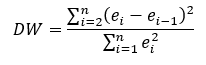where ei is the ith residual from the regression analysis. The value of DW is between 0 and 4. In general, if DW is 2, there is no autocorrelation; 0 to <2 there is positive autocorrelation and >2 to 4 there is a negative correlation. Usually, values under 1 or over 3 are reason for concern about autocorrelation. For the delivery example, DW = 1.17, so autocorrelation is close to being a concern.

PRESS: This is the prediction sum of squares. It measures the difference between observed and fitted values. PRESS is given by the following: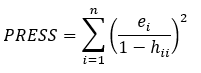where hii is the diagonal element of the hat matrix (H = X(X'X)-1X'). PRESS is a measure of how well the model will predict new values. Lower values of PRESS are desired. PRESS is used to calculate R-squared prediction. In the delivery example, PRESS = 459.0.

R-sq Prediction: This is a measure of the model's ability of predict new observations. Larger R-squared prediction values are desired. The equation for R-squared prediction is: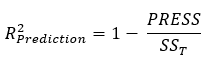The R-squared prediction for the delivery example is 92.06%. This means that the model explains 92.06% of the variability in predicting new observations.

### Summary

This publication is Part 1 of a two-part series on what the regression statistics accompanying linear regression output mean and how they help you understand how valid the model is. This publication looked at the regression model, regression ANOVA table, predictors table and the regression statistics from the SPC for Excel output to help you determine how "good" the regression model is. Part 2 will examine the various types of residuals.

SPC for Excel Software

SPC Training

SPC Consulting

Ordering Information

Thanks so much for reading our publication. We hope you find it informative and useful. Happy charting and may the data always support your position.

Sincerely,

Dr. Bill McNeese
BPI Consulting, LLC#### Connect with Us

•I have enjoyed and appreciated SPC micro for Exel for a long time. It has been the primary tool the quality analysts use in the various healthcare organizations I have assisted. Great product.

Aug 26, 2022
•Thank you for the kind words.  Best Regards, Bill

Aug 30, 2022

### Filtered HTML

• Allowed HTML tags: <a> <em> <strong> <cite> <code> <ul> <ol> <li> <dl> <dt> <dd> <h1> <h2> <h3> <h4> <h5> <h6> <img> <hr> <div> <span> <strike> <b> <i> <u> <table> <tbody> <tr> <td> <th>
• Lines and paragraphs break automatically.

### Plain text

• No HTML tags allowed.
• Lines and paragraphs break automatically.
This question is for testing whether you are a human visitor and to prevent automated spam submissions.

#### SPC Knowledge BaseSign up for our FREE monthly publication featuring SPC techniques and other statistical topics.